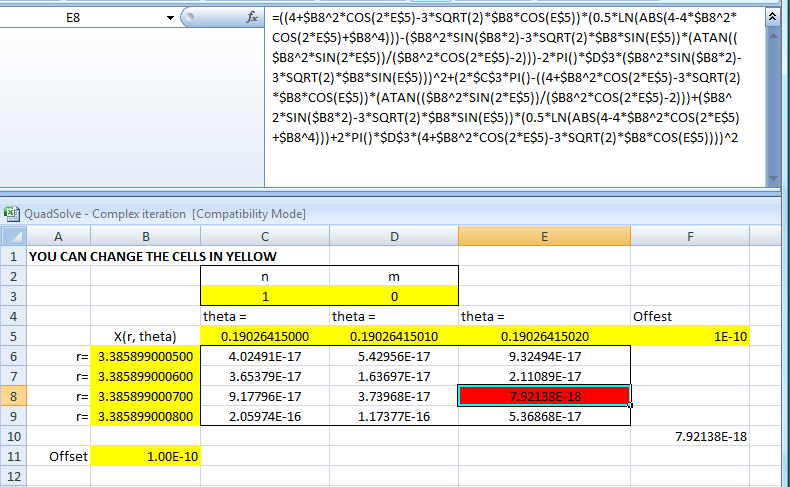#### You may also like### Kissing

Two perpendicular lines are tangential to two identical circles that touch. What is the largest circle that can be placed in between the two lines and the two circles and how would you construct it?### Good Approximations

Solve quadratic equations and use continued fractions to find rational approximations to irrational numbers.### Target Six

Show that x = 1 is a solution of the equation x^(3/2) - 8x^(-3/2) = 7 and find all other solutions.

##### Age 16 to 18 ShortChallenge Level

By applying the quadratic equation formula we can factorise this expression
$$\left(2-x^2\right)^{x^2-3\sqrt{2}x+4} = 1$$
into
$$\left[-(x-\sqrt{2})(x+\sqrt{2})\right]^{(x-\sqrt{2})(x-2\sqrt{2})}=1$$

For real solutions we can make use of the fact that for any real $a\neq 0$ we have
$$a^0 = 1 \quad\quad 1^a=1$$

First look at the base. This equals $1$ if and only if $x=\pm 1$.

Next look at the exponent. This equals zero if and only if $x=\sqrt{2}$ or $2\sqrt{2}$. However, when $x=\sqrt{2}$ both the base and exponent are zero. Therefore, three valid real solutions to the equation are
$$x = \pm 1, x=2\sqrt{2}$$
Is this all? Not necessarily. We might also have $(-1)^{2n}=1$ for any positive whole number $n$.  Are there any solutions to this? $2-x^2 = -1$ has solutions $x=\pm \sqrt{3}$. In this case the exponent becomes $7\pm 3\sqrt{6}$, which is not of the form $2n$. There are therefore no more real solutions.

Note: If we extend to complex numbers then we also have to take into account the fact that there are multiple complex roots of $1$. For example:
$$\left(\frac{-1\pm \sqrt{3}}{2}\right)^3 = 1$$

EXTENSION: READ ON ONLY IF VERY, VERY KEEN!!

Any complex number $z$ can be written in modulus argument form $z=re^{i\theta}$ and the logarithm of a complex number becomes
$$\ln{z} = \ln(re^{i\theta}) = \ln r +\ln(e^{i\theta}) = \ln r + i\theta$$

Using these facts we can attack our problem in the complext numbers. Taking logs of the equations gives

$$(z^2-3\sqrt{2}z+4)\ln(2-z^2)=2n\pi i\quad n\in \mathbb{Z}\quad\quad(\dagger)$$

To progress with the complex logarithm of $(2-z^2)$ it is natural to use modulus argument form of $(2-z^2)$. If we suppose that $z=re^{i\theta}$ then

$$\begin{eqnarray} 2-z^2 &=& 2-r^2e^{2\theta i}\\ &=& 2-r^2\cos 2\theta-ir^2\sin 2\theta\\ &=& \sqrt{(2-r^2\cos 2\theta)^2+(r^2\sin 2\theta)^2} e^{i \tan^{-1}\left(\frac{r^2\sin 2\theta}{r^2\cos 2\theta-2}\right)} \end{eqnarray}$$

Our equation $(\dagger)$ then becomes
$$A(r, \theta) \times B(r, \theta) = 2n\pi i$$
where
$$A(r, \theta)=\left(4+r^2\cos 2\theta -3\sqrt{2} r\cos\theta\right)+i\left(r^2\sin 2\theta-3\sqrt{2}r\sin \theta\right)$$
and
$$B(r, \theta,m)=\frac{1}{2}\ln \left|4-4r^2\cos^22\theta+r^4\right| + i \left[2m\pi +\tan^{-1}\left(\frac{r^2\sin 2\theta}{r^2\cos 2\theta-2}\right)\right]\quad m\in \mathbb{Z}$$
There are no non-real solutions for $n=m=0$ as this excellent piece of mathematics by Stephen Lynch shows (I include his full solution).

However, there might well be solutions for other values of $n$ and $m$. In principle we can attempt to solve this by equating real and imaginary parts for various choices of $n$ and $m$.

Editor's note: further analysis appears to be time consuming, so I looked for numerical answers as follows :

Finding an exact solution would appear to be, well, complex. I simply attempted a numerical solution. To find a numerical solution I looked for small values of the expression
$$X(r, \theta,n,m) = \left|A(r, \theta)B(r, \theta,m)-2n\pi i\right|^2$$
I looked at the case $n=1, m=0$.

I found a solution
$$r = 3.3858990007 \,, \theta = 0.1902641501$$
This solves the equation in the sense that
$$X(3.3858990007 , 0.1902641502,1,0)< 10^{-17}$$

From this numerical exploration is seems likely that other solutions exist, although proof that the iterative scheme used does indeed converge on a genuine solution would require more work. (As an aside it is worth noting that complex numbers enter into all sorts of applied mathematics, such as fluid dynamics and air flow.  In such situations numerical solutions of complex number equations are crucial.)

You might wish to take a look at the a spreadsheet which computes the values $X(r, \theta,n,m)$ for various values.

With numerics there is always the chance of hard to spot error, but here is the input used if you wish to check the logic for yourself (this is a good habit to get into)Forunately $X(2\sqrt{2},0,0,0)=X(1,0,0,0)=X(1,\pi,0,0) = 0$ as we would expect from our analysis of the real case. This sort of check is essential when performing numerics.

Needless to say, if you spot an error please let us know!